NEET  >  Test: Units & Measurement - 1

# Test: Units & Measurement - 1 - NEET

Test Description

## 10 Questions MCQ Test Physics Class 11 - Test: Units & Measurement - 1

Test: Units & Measurement - 1 for NEET 2023 is part of Physics Class 11 preparation. The Test: Units & Measurement - 1 questions and answers have been prepared according to the NEET exam syllabus.The Test: Units & Measurement - 1 MCQs are made for NEET 2023 Exam. Find important definitions, questions, notes, meanings, examples, exercises, MCQs and online tests for Test: Units & Measurement - 1 below.
Solutions of Test: Units & Measurement - 1 questions in English are available as part of our Physics Class 11 for NEET & Test: Units & Measurement - 1 solutions in Hindi for Physics Class 11 course. Download more important topics, notes, lectures and mock test series for NEET Exam by signing up for free. Attempt Test: Units & Measurement - 1 | 10 questions in 10 minutes | Mock test for NEET preparation | Free important questions MCQ to study Physics Class 11 for NEET Exam | Download free PDF with solutions
 1 Crore+ students have signed up on EduRev. Have you?
Test: Units & Measurement - 1 - Question 1

### The pairs of physical quantities that have the same dimensions are

Detailed Solution for Test: Units & Measurement - 1 - Question 1

Reynold's number and coefficient of friction are dimensionless quantities. Curie is the number of atoms decaying per unit time and frequency is the number of oscillations per unit time. Latent heat and gravitational potential both have the same dimension corresponding to energy per unit mass.

*Multiple options can be correct
Test: Units & Measurement - 1 - Question 2

### In the formula X = 3YZ2, X and Z have dimensions of capacitance and magnetic induction respectively. What are the dimensions of Y in MKSQ system ? [JEE-1995,2/100]

Detailed Solution for Test: Units & Measurement - 1 - Question 2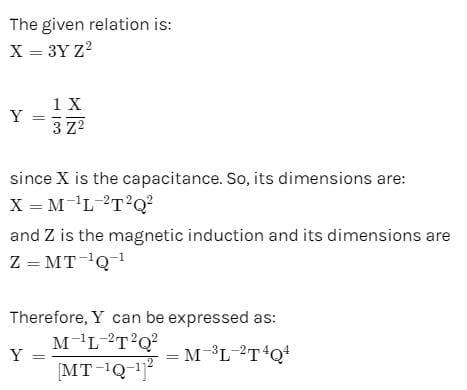Test: Units & Measurement - 1 - Question 3

### Which of the following pairs have same dimensions :

Detailed Solution for Test: Units & Measurement - 1 - Question 3

Torque = Work = Energy =[ML2T-2]

Test: Units & Measurement - 1 - Question 4

The respective number of significant figures for the numbers 23.023, 0.0003 and 2.1 × 10–3 are

Detailed Solution for Test: Units & Measurement - 1 - Question 4

(i) All the non-zero digits are significant.
(ii) All the zeros between two non-zero digits are significant, no matter where the decimal point is, if at all.
(iii) If the number is less than 1, the zero(s) on the right of decimal point but to the left of the first non-zero digit are not significant.
(iv) The power of 10 is irrelevant to the determination of significant figures. According to the above rules, 23.023 has 5 significant figures. 0.0003 has 1 significant figures. 2.1 × 10–3 has 2 significant figures.

Test: Units & Measurement - 1 - Question 5

Let [ε0] denote the dimensional formula of the permittivity of the vaccum, and [μ0] that of the permeability of the vacuum. If M = mass, L = length, T = time and I = electric current :

[JEE-1998' 2/200]

Detailed Solution for Test: Units & Measurement - 1 - Question 5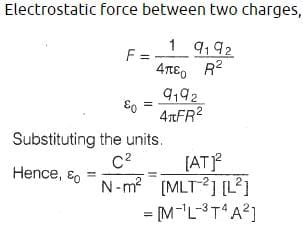Test: Units & Measurement - 1 - Question 6

A dense collection of equal number of electrons and positive ions is called neutral plasma. Certain solids containing fixed positive ions surrounded by free electrons can be treated as neutral plasma. Let 'N' be the number density of free electrons, each of mass 'm'. When the electrons are subjected to an electric field, they are displaced relatively away from the heavy positive ions. If the electric field becomes zero, the electrons begin to oscillate about the positive ions with a natural angular frequency 'wP' which is called the plasma frequency. To sustain the oscillations, a time varying electric field needs to be applied that has an angular frequency w, where a part of the energy is absorbed and a part of it is reflected. As w approaches wP, all the free electrons are set to resonance together and all the energy is reflected. This is the explanation of high reflectivity of metals.
Taking the electronic charge as 'e' and the permittivity as 'ε0', use dimensional analysis to determine the correct expression for wP.

Detailed Solution for Test: Units & Measurement - 1 - Question 6

Dimension of N = [L - 3]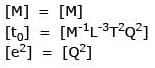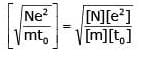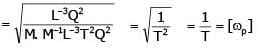Test: Units & Measurement - 1 - Question 7

A quantity X is given by ε0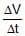. where ε0 is the permittivity of free space, L is length, ΔV is potential difference and Δt is time interval. The dimensional formula for X is the same as that of

[JEE Sc.2000'3/105]

Detailed Solution for Test: Units & Measurement - 1 - Question 7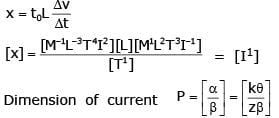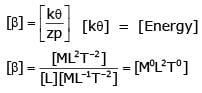Test: Units & Measurement - 1 - Question 8

Pressure depends on distance as, P =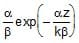, where α, β are constants, z is distance, k is Boltzmann's constant and q is temperature. The dimension of β are

[JEE-2004s '3/84]

Detailed Solution for Test: Units & Measurement - 1 - Question 8

Unit of K is joules per kelvin or the dimensional formula of Kis [ML2T-2θ-1].

αz/kθ should be dimensionless,
So, dimensional formula of α = [ML2T-2]/[L] = MLT-2
Dimensional formula of P = [ML-1T-2]
rArr β = [α/P]

= [MLT-2]/[ML-1T-2]
= M0L2T0

*Multiple options can be correct
Test: Units & Measurement - 1 - Question 9

Which of the following set have different dimensions ?

[JEE-2005s ; 3/60]

Detailed Solution for Test: Units & Measurement - 1 - Question 9

By checking dimension all option
[Dipole moment] = [M0L1T1A1] [Electric Flux] = [M1L3T-3I-1] [Electric field] = [M1L1T-3I-1]

*Answer can only contain numeric values
Test: Units & Measurement - 1 - Question 10

What is the percentage error in the physical quantity A If it is related to four other physical quantities a,b, c and d as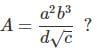The percentage error in measurement of a,b,c and d are 1% , 3% 2% and 1% respectively

Detailed Solution for Test: Units & Measurement - 1 - Question 10

Percentage error of A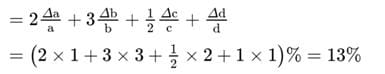## Physics Class 11

130 videos|483 docs|210 tests
Information about Test: Units & Measurement - 1 Page
In this test you can find the Exam questions for Test: Units & Measurement - 1 solved & explained in the simplest way possible. Besides giving Questions and answers for Test: Units & Measurement - 1, EduRev gives you an ample number of Online tests for practice

## Physics Class 11

130 videos|483 docs|210 tests

### How to Prepare for NEET

Read our guide to prepare for NEET which is created by Toppers & the best Teachers(Scan QR code)